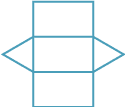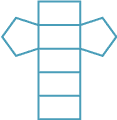#### Question 1

Fill in the blanks to describe the attributes of each prism.

a A rectangular prism has How many faces? faces, How many edges? edges and How many vertices? vertices. A hexagonal prism has How many faces? faces, How many edges? edges and How many vertices? vertices. A triangular prism has How many faces? faces, How many edges? edges and How many vertices? vertices.

#### Question 2

Fill in the blanks to describe the attributes of each prism.

ABCa b c The net for a triangular prism is net: A B C The net for a pentagonal prism is net: A B C The net for an octagonal prism is net: A B C